Before talking about the construction of quadrilaterals, let us recall what a quadrilateral is. A quadrilateral is a polygon that has 4 vertices and 4 sides enclosing 4 angles. The sum of its interior angles is 360 degrees. A quadrilateral, in general, has sides of different lengths and angles of different measures. However, squares, rectangles, etc. are special types of quadrilaterals with some of their sides and angles being equal. In this article, we will discuss the following construction of quadrilaterals:

(i) 4 sides and 1 diagonal is given.

(ii) 3 sides and including 2 angles are given

(iii) 2 sides and three angles are given

## (i) When 4 Sides and One Diagonal are Given

Let us say you are required to construct a quadrilateral PQRS where the measurements are:

• PQ = 5 cm
• QR = 3 cm
• RS = 5 cm
• PS = 4 cm
• Diagonal SQ = 6 cm

For the construction of quadrilaterals with some of the measurements given, we first draw a rough figure of the quadrilateral with the given dimensions, as shown below.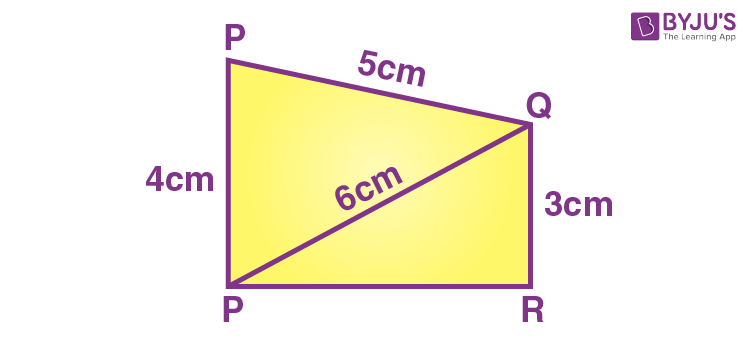Now starting with the construction, the steps are:

• Draw a line segment of length 5 cm and mark the ends as S and R.• Set your compass to the radius of 3 cm and make an arc from the point R above the line segment.
• Set the compass to the radius of 6 cm and make an arc from the point S on the previous arc.
• Mark the point as Q where the two arcs cross each other. Join the points S and Q as well as R and Q.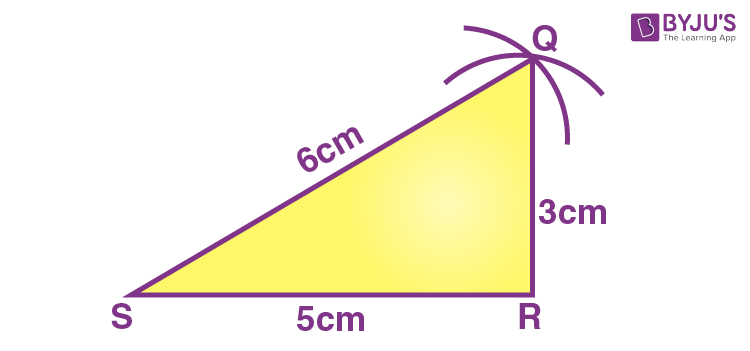• Set the compass to the radius of 5 cm and make an arc from the point Q.
• Set the compass to the radius of 4 cm and make an arc from the point S on the previous arc.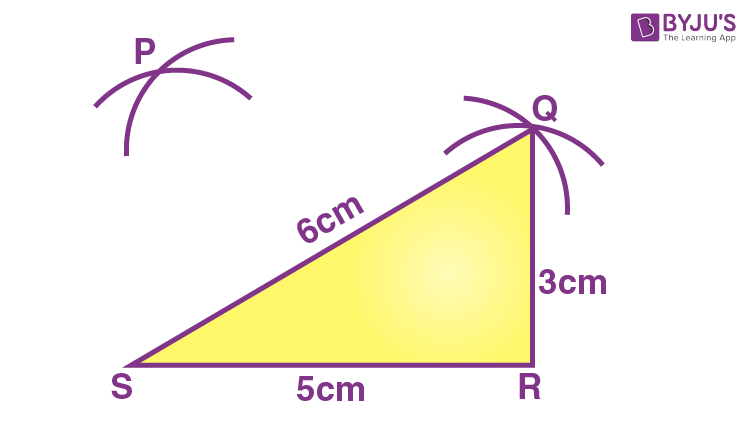• Mark the point as P where the two arcs cross each other.
• Join the points P and Q as well as P and S.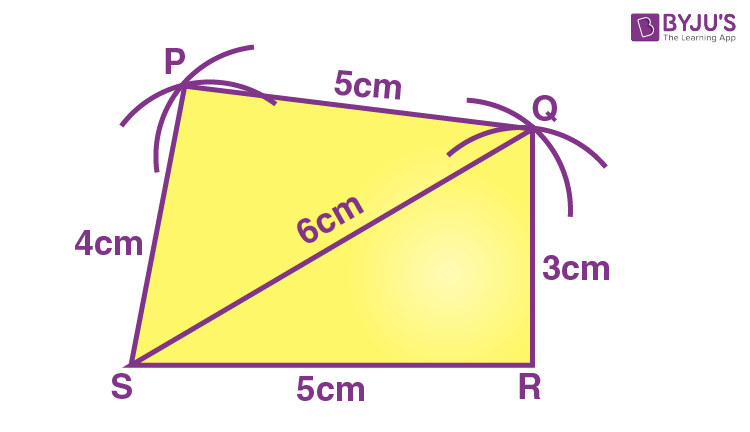You obtain the quadrilateral PQRS of the required measurements.

## (ii) When 3 Sides and Including 2 Angles are Given

Let us say you are required to construct a quadrilateral PQRS where the measurements are:

• QR = 6 cm
• RS = 5 cm
• PS = 4 cm
• ∠S = 100 degrees
• ∠R = 120 degrees

For the construction of quadrilaterals with some of the measurements given, we first draw a rough figure of the quadrilateral with the given dimensions, as shown below.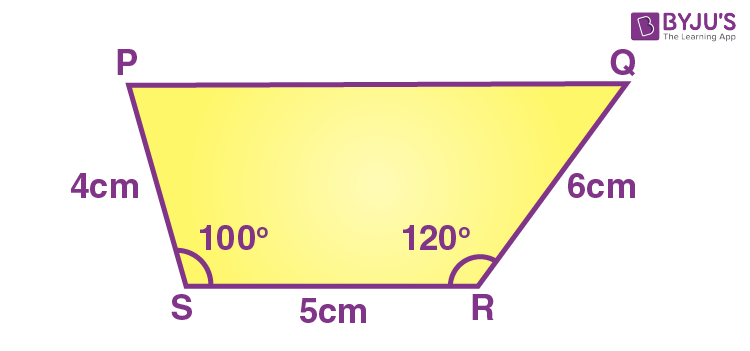Now starting with the construction, the steps are:

Step 1: Draw a line segment of length 5 cm and mark the ends as S and R.Step 2: Using a protractor, draw a line from point R making 120 degrees and another line from the point S making 100 degrees with the line segment SR.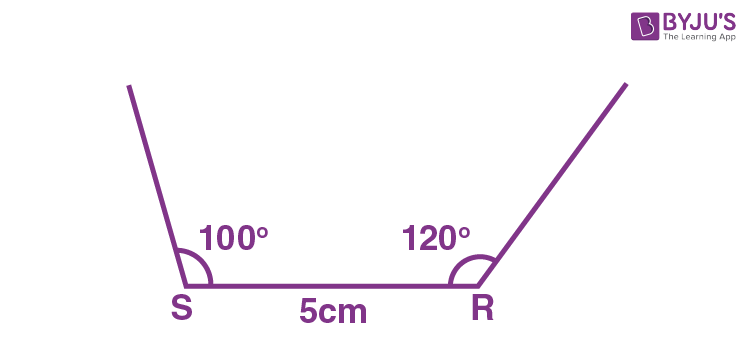Step 3: Set your compass to the radius of 4 cm and make an arc from the point S on the 100-degree line. Mark the point as P where the arc intersects the line.

Step 4: Similarly, set the compass to the radius of 6 cm and make an arc from point R on the 120-degree line. Mark the point as Q where the arc intersects the line.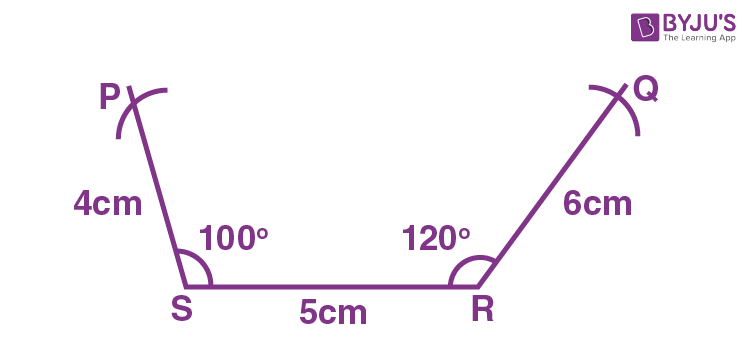Step 5: Join the points P and Q.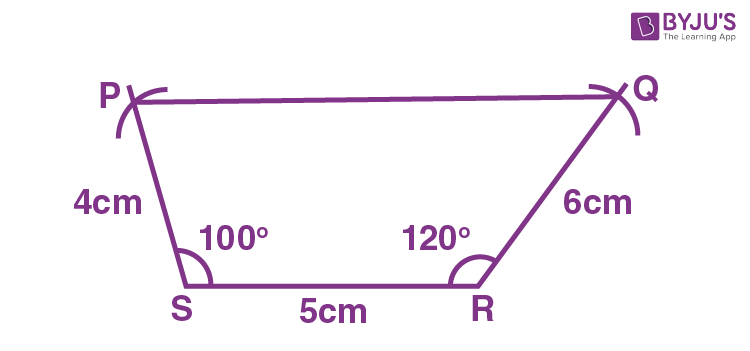You obtain the quadrilateral PQRS of the required measurements.

## (iii) When 2 Sides and Three Angles are Given

Let us say you are required to construct a quadrilateral ABCD where the measurements are:

• AB = 5 cm
• BC = 3 cm
• ∠A = 120 degrees
• ∠B = 110 degrees
• ∠C = 90 degrees

The steps for the construction of the quadrilateral ABCD are:

Step 1: Draw a line segment of length 5 cm and mark the ends as A and B.Step 2: Using a protractor, draw a line from the point A making 120 degrees with the line segment AB.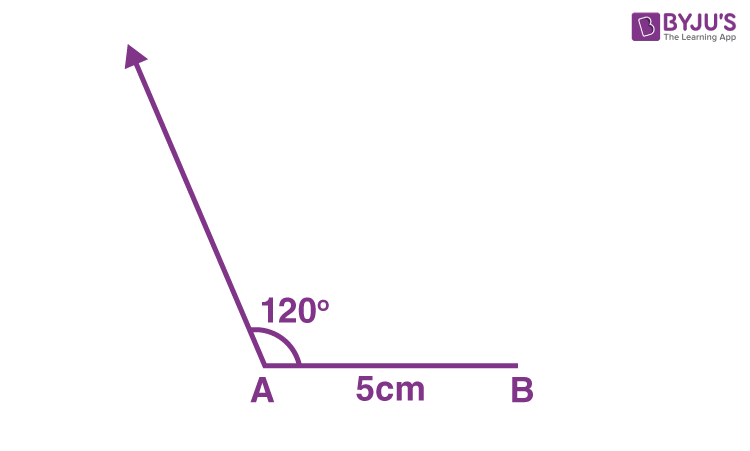Step 3: Using the protractor, draw a line from point B making 110 degrees with the line segment BA.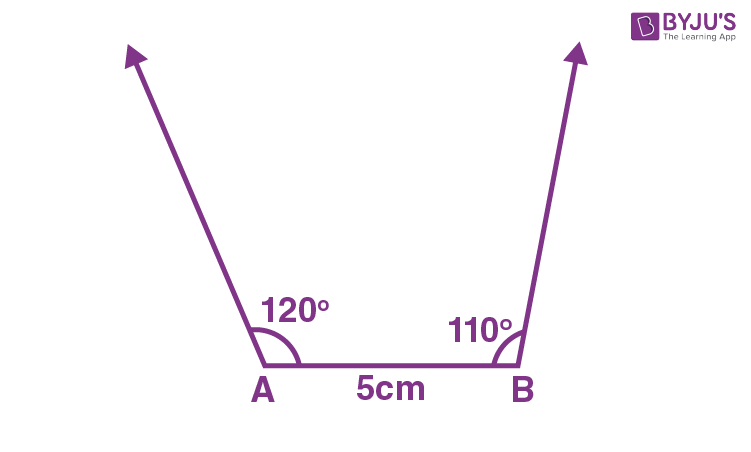Step 4: Set your compass to the radius of 3 cm and make an arc from point B on the 110-degree line. Mark the point as C where the arc intersects the line.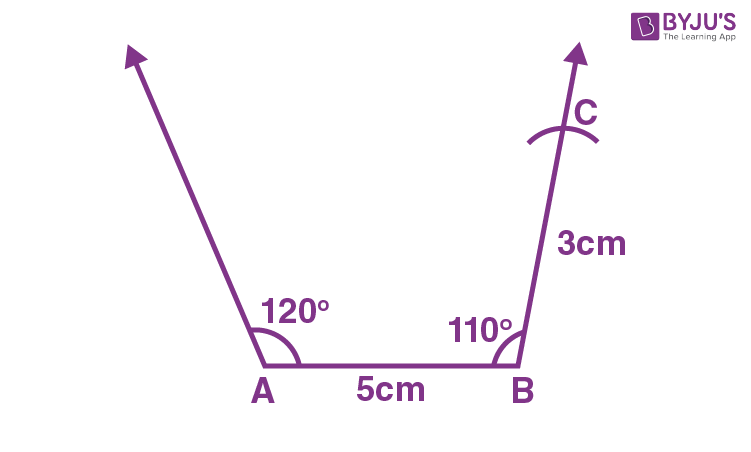Step 5: Using the protractor, draw a line from point C making 90 degrees with the line segment CB. Mark the point as D where the arc intersects the 120-degree line.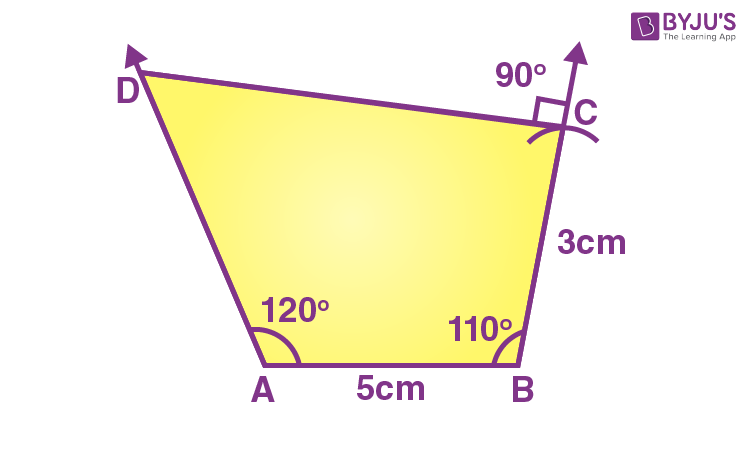You obtain the quadrilateral ABCD of the required measurements. Since the sum of the interior angles of a quadrilateral is 360 degrees, you can check the measure of ∠D which should be equal to 40 degrees (360 – [120 + 110 + 90]).